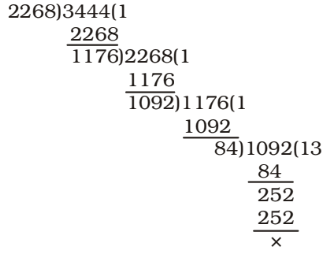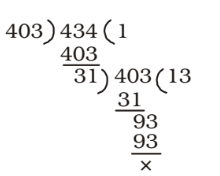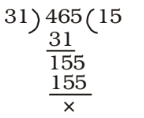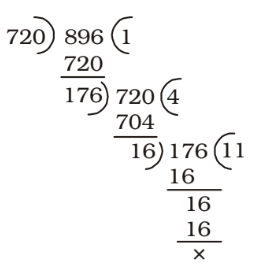## LCM and HCF

#### LCM and HCF

1. The greatest number by which 2300 and 3500 are divided leaving the remainders of 32 and 56 respectively, is

1. As we know that the largest number which when divide the numbers a, b and c give remainders as p, q, r respectively is given by H.C.F. of (a – p), (b – q) and (c – r).
Required number = HCF of 2300 – 32 = 2268 and 3500 – 56 = 3444##### Correct Option: D

As we know that the largest number which when divide the numbers a, b and c give remainders as p, q, r respectively is given by H.C.F. of (a – p), (b – q) and (c – r).
Required number = HCF of 2300 – 32 = 2268 and 3500 – 56 = 3444∴ HCF = 84
Hence , Required number = 84

1. There are 24 peaches, 36 apricots and 60 bananas and they have to be arranged in several rows in such a way that every row contains the same number of fruits of only one type. What is the minimum number of rows required for this to happen ?

1. Minimum number of rows = Maximum number of fruits in each row
∴ HCF of 24, 36 and 60 = 12

 ∴ Minimum number of rows = 24 + 36 + 60 12 12 12

##### Correct Option: C

Minimum number of rows = Maximum number of fruits in each row
∴ HCF of 24, 36 and 60 = 12

 ∴ Minimum number of rows = 24 + 36 + 60 12 12 12

Hence , Minimum number of rows = 2 + 3 + 5 = 10

1. Three tankers contain 403 litres, 434 litres, 465 litres of diesel respectively. Then the maximum capacity of a container that can measure the diesel of the three containers exact number of times is

1. Greatest capacity of measuring vessel
= HCF of 403 litres, 434 litres and 465 litres
First of all we find HCF of 403 and 434Again , HCF of 31 and 465##### Correct Option: A

Greatest capacity of measuring vessel
= HCF of 403 litres, 434 litres and 465 litres
First of all we find HCF of 403 and 434Again , HCF of 31 and 465∴ Greatest capacity of measuring vessel = 31 litres

1. The greatest number that will divide 729 and 901 leaving remainders 9 and 5 respectively, is

1. We know that the largest number which when divide the numbers a, b and c give remainders as p, q, r respectively is given by H.C.F. of (a – p), (b – q) and (c – r).
Required number = HCF of (729 – 9) = 720 and (901 – 5) = 896H.C.F = 16

##### Correct Option: B

We know that the largest number which when divide the numbers a, b and c give remainders as p, q, r respectively is given by H.C.F. of (a – p), (b – q) and (c – r).
Required number = HCF of (729 – 9) = 720 and (901 – 5) = 896H.C.F = 16
Hence , required answer = 16.

1. 84 Maths books, 90 Physics books and 120 Chemistry books have to be stacked topic wise. How many books will be there in each stack so that each stack will have the same height too ?

1. As the height of each stack is same,
The required number of books in each stack = HCF of 84, 90 and 120
84 = 2 × 2 × 3 × 7
90 = 2 × 3 × 3 × 5
120 = 2 × 2 × 2 × 3 × 5
∴ HCF = 2 × 3 = 6

##### Correct Option: C

As the height of each stack is same, the required number of books in each stack
= HCF of 84, 90 and 120
84 = 2 × 2 × 3 × 7
90 = 2 × 3 × 3 × 5
120 = 2 × 2 × 2 × 3 × 5
∴ HCF = 2 × 3 = 6
Hence , The required number of books in each stack is 6 .